MacKenzie, I. S. (2013). A note on the validity of the Shannon formulation for Fitts' index of difficulty. Open Journal of Applied Sciences, 3(6), 360-368. [PDF]

# A Note on the Validity of the Shannon Formulation for Fitts' Index of Difficulty

I. Scott MacKenzie
Dept. of Computer Science and Engineering
York University
Email: mack@cse.yorku.ca

ABSTRACT: The three most common variations of Fitts' index of difficulty are the Fitts formulation, the Welford formulation, and the Shannon formulation. A recent paper by Hoffmann  critiqued the three and concluded that the Fitts and Welford formulations are valid and that the Shannon formulation is invalid. In this paper, we challenge Hoffmann's position regarding the Shannon formulation. It is argued that the issue of validity vs. invalidity is ill-conceived, given that Fitts' law is a "model by analogy" with no basis in human motor control. The relevant questions are of utility: Does a model work? How well? Is it useful? Where alternative formulations exist, they may be critiqued and compared for strengths and weaknesses, but validity is an irrelevant construct. In a re-analysis of data from Fitts' law experiments, models built using the Shannon formulation are (re)affirmed to be as good as, and generally better than, those built using the Fitts or Welford formulation.

Keywords: Fitts' law, index of difficulty, Shannon formulation, effective target width, scientific method

## 1. Introduction

Scientists pursue their research using a body of techniques known as the scientific method. Ideas are framed as hypotheses that challenge conventional wisdom about the physical world. The goal is discovery. The method is empirical: Observations are made, measurements are taken, evidence is gathered. Hypotheses are tested against evidence and conclusions are drawn. While strong evidence yields strong conclusions, hypotheses are never proven. That's the nature of science. Proof lies within the realm of mathematics – the study of numbers, including their relationships, operations, structure, and so on. If a numeric relationship is proposed and subsequently demonstrated by analysis to violate the allowed and correct operations, the relationship is deemed invalid. Validity or invalidity is an inherent dichotomy, established through analysis. There is no need for empirical evidence to support the case.1

In this paper, we examine Hoffmann's claim that the Shannon formulation for Fitts' index of difficulty is invalid, and that the Fitts and Welford formulations are valid. Along the way, deficiencies in his analysis and a few errors are noted. As inferred in the preceding paragraph, we will touch on issues pertaining to the methodology in scientific inquiry, such as the roles of analysis and empirical evidence and the demand for rigor and due diligence. We will also reach into a rather unique property of Fitts' law: Fitts' law is a model by analogy, with no basis in human motor control. Because of this, we argue that validity is an ill-conceived and meaningless construct. The only test is utility. On this point, there is ample evidence – both old and new – that the Shannon formulation works as wells as, and generally better than, the Fitts or Welford formulation.

This paper is organized in the three parts: background, analysis, evidence. In the first part, we provide background discussion on Fitts' law, emphasising issues relevant to Hoffmann's analysis and the contrary points developed herein. The discussion is not a thorough review of Fitts' law. For that, the reader is directed to a few published sources which are also available online [2-4]. In the second part, we present and critique the analysis developed by Hoffmann on the validity and invalidity of the formulations. Hoffmann's position is built on a presumption that human movements are equivalent to electrical signals. As no such equivalence exists, we demonstrate that Hoffmann's arguments, while perhaps interesting, are meaningless and futile to his purpose. The third section examines the empirical evidence Hoffmann offers in reanalysing published data. While such analyses are common and often provide insight, they are irrelevant on the question of validity vs. invalidity for alternative formulations of Fitts' index of difficulty. Nevertheless, deficiencies in the analyses are noted. We conclude with an analysis of a recently published data set. The analysis reaffirms the utility of the Shannon formulation for Fitts' index of difficulty.

## 2. Background

Like many psychologists in the 1950s, Fitts was motivated to investigate whether human performance could be quantified using a metaphor steeped in the new and emerging language of information theory. Fitts' particular interest was rapid-aimed movements, where a human operator acquires or selects targets of a certain size over a certain distance. Fitts proposed a model – now law – that is widely used in fields such as ergonomics, engineering, psychology, and human-computer interaction [5, 6]. The starting point for Fitts' law is an equation known as Shannon's Theorem 17, which appears on the first page of Fitts' influential paper [6, p. 381].2 The equation gives the information capacity C (in bits/s) of a communications channel of bandwidth B (in s-1 or Hz) as

C = B log2(S / N + 1)          (Eq. 1)

where S is the signal power and N is the noise power [8, pp. 100-103]. Fitts reasoned that a human operator that performs a movement over a certain amplitude to acquire a target of a certain width or tolerance is demonstrating a "rate of information transfer" [6, p. 381]. In Fitts' analogy, movement amplitudes are like signals and target tolerances or widths are like noise.

Fitts proposed an index of difficulty (ID) for a target acquisition task using a log-term slightly rearranged from Eq. 1. Signal power (S) and noise power (N) are replaced by movement amplitude (A) and target width (W), respectively:

ID = log2(2A / W)         (Eq. 2)

As with the log-term in Eq. 1, the units are bits because the ratio within the parentheses is unitless and the log is taken to base 2. The source Fitts cited in introducing his formulation used a version of Shannon's Theorem 17, with the +1 removed [6, p. 388, 7, p. 157]. Although a convenient simplification, it was noted that the formulation should only be used if the signal-to-noise ratio is large. But, the A:W ratio in Fitts' law experiments is often as low as 1:1. Fitts prefixed A with 2 because it "ensures the index will be greater than zero for all practical situations" [6, p. 388].

Several variations of ID have been proposed over the years. Of relevance here are the Welford formulation [9, p. 147]:

ID = log2((A + 0.5W) / W ) = log2(A / W + 0.5)          (Eq. 3)

and the Shannon formulation :

ID = log2(A / W + 1.0)          (Eq. 4)

The Welford formulation was proposed on practical terms: "[the subject] is called upon to choose a distance W out of a total distance extending from his starting point to the far edge of the target" [9, p. 147]. Going from the center of the target to the far edge adds 0.5W to A, which is revealed in the first form of ID in Eq. 3. Welford dropped the 2 in Fitts' formulation because, as he noted, "the logarithm can never be negative, since in the extreme case when the movement begins at the edge of the target A = ½W " [9, p. 147].

The Shannon formulation (Eq. 4) was proposed to create a direct analogy with Shannon's Theorem 17 (Eq. 1). MacKenzie proffered that there was no strong case given by Fitts or Welford to deviate from the arrangement of terms in Shannon's theorem. If the goal is to measure "the information capacity of the human motor system" (the title of Fitts' 1954 paper), then it is reasonable to arrange the terms in direct correspondence with Shannon's theorem.

The Shannon formulation is also appealing in that ID smoothly approaches 0 bits as A approaches 0. This is seen in Figure 1, contrasted with the Fitts and Welford formulations which dip negative for small A. Although a negative ID is unlikely in most situations, there are at least four examples of ID < 0 in the Fitts' law literature [11-14]. With the Shannon formulation, ID < 0 is simply not possible.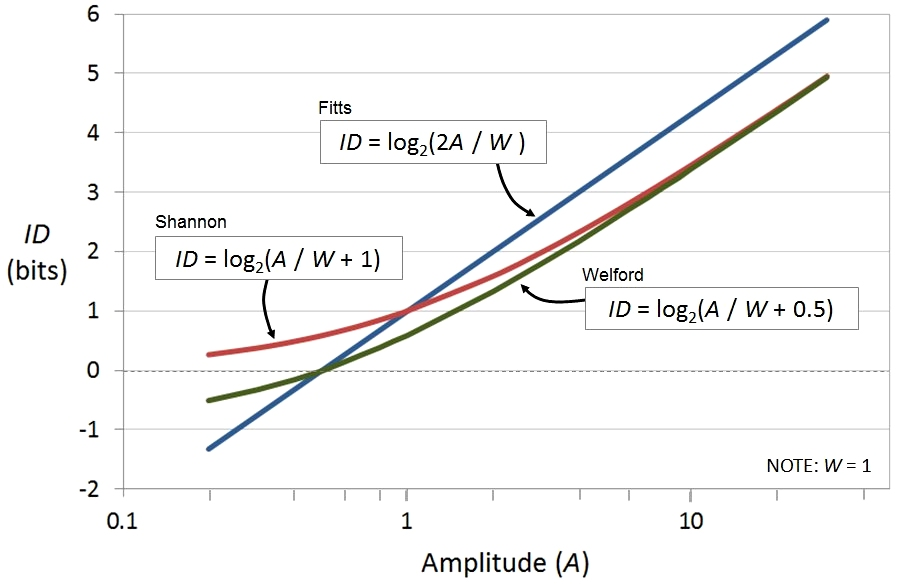Figure 1. With the Shannon formulation, ID approaches 0 as A approaches 0.

Note in Figure 1 that the lines are nearly parallel except when ID is small. This is an important point. Quantitative analyses seeking to distinguish the three formulations must attend to the range of IDs. Only where the range includes low values of ID are differences likely to emerge.3 We will return to this point later.

Fitts described three experiments in his 1954 paper. The first involved reciprocal tapping of targets with either a 1-oz or a 1-lb stylus. Four amplitudes and four widths were used, yielding 16 target conditions. Fortunately, Fitts published summary data tables so a re-examination of his results is possible. The data for the 1-oz stylus condition are given in Table 1, and include target amplitude (A), target width (W), error rate (ER), index of difficulty (ID), and movement time (MT). The effective target width (We) column was added, as discussed shortly.

Table 1
Data From Fitts' Tapping Experiment With 1-oz StylusFitts conjectured that the MT-ID relationship is approximately linear, implying a constant rate of information processing. This is reasonably confirmed in the scatter plot and linear regression analysis in Figure 2. With R2 = .9664, the model explains 96.6% of the variance in the data – a good fit, indeed. Nevertheless, there is a curving of data points away from the regression line, with the most deviate point at ID = 1 bit (see block arrow). Crossman first pointed this out in 1957 in an unpublished report [cited in 9, p. 146].4 Similar observations and analyses were provided by Welford  shortly after. Both Crossman and Welford sought to improve the model. Welford's approach was a new formulation for ID, as given above in Eq. 3. Crossman's approach was quite different.Figure 2. Scatter plot and regression line for data in Table 1. See text for discussion.

Crossman sought to improve the information-theoretic analogy in Fitts' law by replacing the specified or set target width (akin to noise) by an effective target width that reflects the spatial variability in the human operator's responses over repeated trials. Welford succinctly paraphrases Crossman's method thus:

[The method] makes use of the fact that the information in a normal distribution is log2((2 π e)½ × σ), where σ is the standard deviation in a normal distribution. Now (2 π e)½ = 4.133 and a range of ± half this, i.e., 2.062 σ, includes about 96% of a normal distribution. We can therefore argue that if about 4% of the shots fall outside the target, log2W is an accurate representation of the information contained in the distribution of shots. We can argue that if the errors exceed 4% the effective target width is greater than W, and if the errors are less than 4% the effective target width is less than W. How much greater or less can be calculated from tables of the normal distribution. For example, suppose W = 2 and the errors are 1%. Then the effective W = 2 × 4.133 / 5.152 = 1.604 in, since all but 1% of a normal distribution lie within a range of ±2.576 (i.e., ½ × 5.152) of the mean. [9, pp. 147-148]5

Although Welford sought to improve the fit of the model – bring the scatter points closer to the best-fitting line – Crossman's change has an even more important consequence: If the model is built using the effective target width (We), Fitts' law truly embeds the speed-accuracy trade-off.

The technique described above to determine the effective W is known as the discrete-error method since it uses the error rate and z-scores from a unit-normal distribution in transforming W. An alternative method is the standard-deviation method. If the experimental apparatus records the coordinates of selection for each trial, then the standard deviation (σ) is computed directly, with We = 4.133 σ. Obviously, the standard-deviation method is preferred since the transformation is more sensitive to the actual spatial variability in responses.

The apparatus in Fitts' experiment recorded "hits" and "misses", thus the error rate (ER) as a percentage was easily obtained. The apparatus did not record selection coordinates. The We column in Table 1 was developed from the ER column using the discrete-error method, as described by Welford. The first entry poses a problem, however, since the task was easy (ID = 1 bit) and no misses were recorded. The We value was developed using a pragmatic approach. Fitts reported the error rate for the top row as "0.00%". This was converted to "0.0049%", which rounds to 0.00%, with the z-score obtained thus [3, p. 108]. Although not explicitly stated, Welford likely used a similar heuristic since the point corresponding to ID = 1 bit appears in his reanalysis of Fitts' data using effective target widths. This is presented next.

If the data in Table 1 are plotted as in Figure 2, except using the Welford formulation or using the effective target width, the fit of the model is indeed improved. This is evident in Figure 3a in which both Welford's ID formulation and Crossman's adjustment for accuracy are applied. Welford presented a chart that is essentially the same, with following observation: "the results lie close to a straight line which passes through the origin" [9, pp. 148-149]. Indeed, the correlation is very good (R2 = .9885) and the intercept is very small (1.22 ms).6

(a)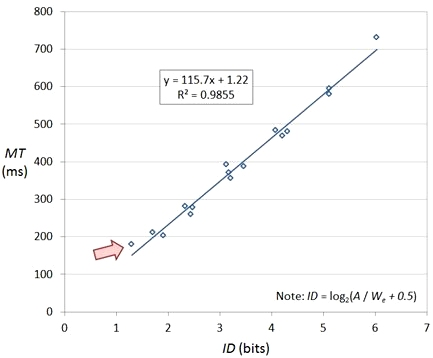(b)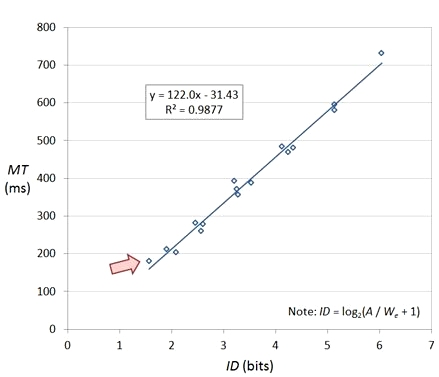Figure 3. Scatter plot and regression analysis using data from Fitts' tapping experiment with a 1-oz stylus. Both charts use the effective target width. (a) Welford formulation. (b) Shannon formulation. Source data: Table 1.

Using the Shannon formulation (see Figure 3b), there is a slight improvement in the fit (R2 = .9877), although the intercept is larger (-31.43 ms). Importantly, the charts in Figure 3 contain 16 scatter points. Note that the point identified by the block arrow is now much closer to the best-fitting line. Including this condition is important, since (a) it was the most deviate point in the original analysis, and (b) low values of ID are needed to distinguish the different formulations of ID, as demonstrated earlier (see Figure 1).7

## 3. Analysis

Hoffmann's analysis leading to the conclusion that the Shannon formulation for Fitts' index of difficulty is invalid hinges on two points: "movements are not a continuous signal" [1, p. 210] and there is "as incorrect substitution of an amplitude in place of a signal power" [1, p. 213].

It is certainly true that movement amplitude in Fitts' law is substituted for signal power in Shannon's Theorem 17. Whether this is incorrect is a matter for debate, which we get to shortly. Hoffmann expounds on the possibility and mechanisms for using a more power-like variation of movement amplitude in Fitts' law. The discussion is interesting and might very well suggest a new formulation for Fitts' index of difficulty. But that is a separate issue (and, we might add, an issue in need of empirical evidence).

Hoffmann's invalidity claim is deficient in at least two ways. We preface the first with observations on Fitts' law and modeling in general. Most models are developed from within a discipline: Low-level established principles are used to explain higher-level phenomena. In human-computer interaction, the best-known example is the keystroke-level model (KLM) introduced more than 30 years ago , and still widely used today. With the KLM, the low-level principles are primitive actions such as the keystroking time for commands, mouse-to-keyboard homing time, and so on. High-level phenomena are actions like search-and-replace, file copy, delete a paragraph, etc. The KLM is a model developed from within the discipline. Most models can be characterised similarly. Not so, with Fitts' law. Fitts' law is a model by analogy, with no basis in human motor control. The model uses low-level established principles in electronic communications. But, the phenomena of electronic signals exist in far-off world from the phenomena of human movements. Because of this, the correctness of Fitts' law – or any such model by analogy – cannot be established through analysis. One might postulate that movement amplitude is like a signal or that target width is like a noise distribution, but there is no mathematical or analytic basis to deem the is-like-a link between the two worlds correct, incorrect, valid, invalid, or whatever. The only choice is to the test the model empirically – to weigh observations against predictions.

The first deficiency in Hoffmann's analysis is the presumed equivalence of the phenomena of electronic communications systems with those of human movement. Of course, no such equivalence exists: Human movements are not electronic signals – in any form. Because the link is by analogy, it is irrelevant whether the signal in Shannon's theorem is peak or power, discrete or continuous, filtered or unfiltered, etc. Validity or invalidity is simply the wrong construct. The issue is utility, not validity. Aside from that, the Fitts and Welford formulations use the same measure of movement amplitude and in exactly the same way – in the numerator of the log-term. This point is examined next.

The second deficiency in Hoffmann's argument is his opposing and incompatible positions on the Shannon formulation (invalid) and the Welford formulation (valid). His claim is perplexing since the two formulations differ only in the use of +1.0 (Shannon) vs. +0.5 (Welford) in the log-term. Why would one version be valid, the other invalid? Welford's rationale for +0.5 was to add the distance from the center of the target to the far edge, which is 0.5 × W. MacKenzie's rationale for +1.0 was simply that this is the arrangement in Shannon's Theorem 17. If the rationale for the Shannon formulation was different, would that matter? What if MacKenzie said nothing about the Shannon formulation, but simply argued to change Welford's +0.5 to +1.0 because of the desirable property that +1.0 yields ID = 0 bits when A = 0? Let's call this the Plus-one formulation. It is identical to the Shannon formulation. So we ask: Is the Plus-one formulation valid? On what basis would Hoffmann deem the Plus-one formulation invalid?

Clearly, there is no argument on the basis of signal power or continuous signals. Once again, we see that validity vs. invalidity is an ill-conceived construct. The only issue is utility: Do the formulations work? Which one provides a better descriptive or predictive ability to explain human responses for rapid-aimed movements?

## 4. Evidence

Data from three sources were analysed by Hoffmann and offered as evidence for his position that the Fitts and Welford formulations for ID are valid and that the Shannon formulation is invalid.

Although we already noted the irrelevance of empirical evidence in view of an analytic determination of invalidity, let's examine Hoffmann's evidence to see what insights are offered. Once again, we find deficiencies. Two data sets analysed by Hoffmann have already been dealt with, and are not discussed further (see footnotes 3 and 7). The third data set is from a paper publish by MacKenzie in 1995. Let revisit Hoffmann's reanalysis.

First, it is worth noting that the 1995 paper cited is not a research paper. It is a review paper with a pedagogical intent. An example data table was used to illustrate applications of Fitts' law. The table is a subset of a table from MacKenzie , which is cited in the 1995 paper and which has been available online since the mid-1990s. By using a partial data set, Hoffmann's analysis is incomplete.8 The results of Hoffmann's analysis are given in Figure 4. There are six Fitts' law models. The top three use set target widths (W), the bottom three use effective target widths (We). Within each group, there are models for the Fitts, Welford, and Shannon formulations. Reflecting on Figure 4, Hoffmann notes, "there is a marked reduction in the correlation when both the effective target width and the Shannon formulation are used in the regression" [1, p. 212].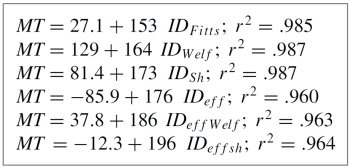Figure 4. Hoffmann's reanalysis of data from MacKenzie (1995).

There are at least three problems in Hoffmann's analysis. First, his observation is simply wrong. Yes, there is a reduction in the three correlations using the effective target widths ("eff" in the figure).  This effect is well known [e.g., 20, p. 479]. However, with respect to the Shannon formulation, his observation is wrong.  In fact, the opposite is true. The correlations within each group are highest using the Shannon formulation.

Second, Hoffmann did not bring the same standard of rigor to the analysis as used in the paper he sought to criticize. In MacKenzie's  comparison of the Fitts, Welford, and Shannon formulations, correlations were computed and a statistical significance test was used to determine if the differences were significant. Hoffmann included no such test. An appropriate test is Hotelling's t-test for the correlations of correlated samples [e.g., 21, p. 164].

Third, Hoffmann did not exercise due diligence to obtain and use the original and complete data set for his analysis, even though the source is cited and is readily available online.9 Hoffmann excluded the data point for ID = 1 bit because the example data table only included error rates and the error rate was 0.0% at ID = 1 bit (E. R. Hoffmann, personal communication, June 19, 2013). As noted earlier (see Figure 1), it is with low values of ID that the distinction between the three formulations emerges. So, to needlessly exclude this data point in a critical analysis that seeks to compare the three formulations falls short of the standards of rigor demanded in the analysis. The complete data set is given in Table 2 and includes a column labeled We(SD) for the effective target width as computed using the standard deviation in the selection coordinates. With this, it is easy to compute the effective index of difficulty for all 16 data points. For convenience, six columns are included showing ID computed using the Fitts, Welford, and Shannon formulations using set target widths (W) and effective target widths (We).

Table 2
Data From MacKenzie (1995) With an Additional Column for We(SD).Hoffmann's analysis is repeated in Table 3, using the data in Table 2. The ranking of correlations within each target width is Fitts (lowest), Welford (middle), Shannon (highest). So, the results are favourable to the Shannon formulation. However, the differences in correlations are modest. Hotelling's t-test deemed the difference between the Fitts and Shannon correlations not significant both using set targets widths (t16 = 1.29, p > .05) and using effective target widths (t16 = 0.34, p > .05 ).10

Table 3
Fitts' Law Models and Correlations Using the
Fitts, Welford, and Shannon Formulations for ID Using Set and Effective Target Widths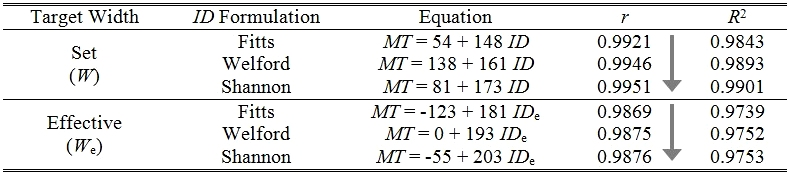The results in Table 3 are not dramatically different from those in Figure 4. The purpose here is the do the analysis correctly: using the full data set, employing an acceptable standard of rigor, and drawing correct conclusions.

One final point about the analysis in Table 3 will be made. The correlations are lower for the models using the effective target width (We) compared to those using the set target width (W). This is a natural consequence of the reduced range of IDs when computed using the effective target width. Note, for example, that the ID range in the Fitts-W column in Table 2 is 7 – 1 = 6 bits, whereas the ID range in the Fitts-We column is 6.620 – 1.988 = 4.633 bits. The lower correlations in the latter case are much like the statistical effect known as "regression toward the mean." It is important to remember that the benefit in using the effective target width is not because it produces a model with higher correlations (although this sometimes occurs, see Figure 2 and Figure 3), but, rather, it brings accuracy into Fitts' law, and makes it a true speed-accuracy model of human motor behavior. Further discussion on this is provided by Soukoreff and MacKenzie [4, section 3.2].

## 5. A Modern Example

Since the Shannon formulation was introduced in 1989, it has been generally accepted as the preferred formulation for Fitts' law. This is particularly the case in human-computer interaction (HCI), where there is an active community of researchers exploring and pushing the limits of Fitts' law. For the most part, there is no debate on which formulation to use. Other issues are considered more interesting, such as applying Fitts' law in 3D virtual environments , using Fitts' law for touchscreen input where fingers select small targets , or examining if input control using device tilt can be modeled by Fitts' law . The Shannon formulation is generally the formulation of choice.

Of course, the analysis above can be pursued with other data sets, provided summary data are published or are available first-hand. One recent example is a data set for an experiment comparing a mouse and a gyroscope-based remote pointer [25, p. 253]. The data set is in the same format as in Fitts' original publication, with an additional column for the effective target width (We). With such data, it is easy to compare ID formulations, as a demonstrated above. See Table 4. There are four tests: 2 devices × 2 methods of calculating target widths. In all four cases, the rank of correlations is Fitts (lowest), Welford (middle), Shannon (highest), although the differences are modest.

Table 4
Comparison of ID Formulations in a
Fitts' Law Experiment Comparing a Mouse With a Remote Pointer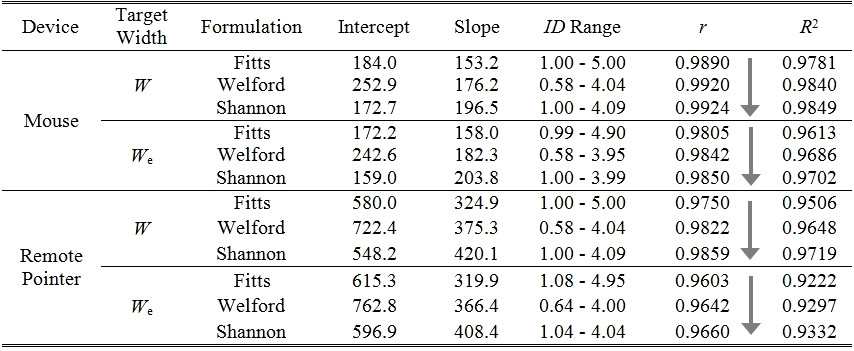Comparing by target width in Table 4, the correlations are consistently lower with the effective target width (We) vs. the set target width (W). To help illustrate why, an extra column is added showing the ID range for each model. The range varies due the inherent differences in the ID formulations and to the method of calculating target widths, as noted above. For all 6 formulation × device comparisons, the range is less using We compared to W. For example, the ID range in the top row is 5.00 – 1.00 = 4.00 bits, corresponding to the Mouse-W-Fitts model. The range for the Mouse-We-Fitts model (three rows down) is 4.90 – 0.99 = 3.91 bits. The lower ID using We is simply an artefact of one's choice to include accuracy in the Fitts' law model.

## 6. Conclusion

We have examined Hoffmann's claim that the Shannon formulation for Fitts' index of difficulty is invalid. Several deficiencies in his analysis were noted. We have argued that because Fitts' law is a model by analogy, there is no analytic basis on which to deem the Shannon formulation (or any other formulation) valid or invalid. The only test is utility, which demands empirical evidence. Hoffmann's empirical evidence (although irrelevant to the question of invalidity) was also examined. Again, deficiencies were noted, such an erroneous observation, the use of an incomplete data set (when the full data set is available), and the failure to exercise the same standard of rigor as used in the research where the Shannon formulation was originally introduced. In a proper reanalysis using the full data set and in an analysis of a recently published data set, the Shannon formulation is reaffirmed to provide better predictions than the Fitts or Welford formulation.

## REFERENCES

  E. R. Hoffmann, "Which Version/Variation of Fitts' Law? A Critique of Information-Theory Models," Journal of Motor Behavior, Vol. 45, 2013, pp. 205-215.  I. S. MacKenzie, "Fitts' Law as a Performance Model in Human-Computer Interaction", Doctoral Dissertation, University of Toronto (http://www.yorku.ca/mack/phd.html), 1991.  I. S. MacKenzie, "Fitts' Law as a Research and Design Tool in Human-Computer Interaction," Human-Computer Interaction, Vol. 7, 1992, pp. 91-139.  R. W. Soukoreff and I. S. MacKenzie, "Towards a Standard for Pointing Device Evaluation: Perspectives on 27 Years of Fitts' Law Research in HCI," International Journal of Human-Computer Studies, Vol. 61, 2004, pp. 751-789.  P. M. Fitts and J. R. Peterson, "Information Capacity of Discrete Motor Responses," Journal of Experimental Psychology, Vol. 67, 1964, pp. 103-112.  P. M. Fitts, "The Information Capacity of the Human Motor System in Controlling the Amplitude of Movement," Journal of Experimental Psychology, Vol. 47, 1954, pp. 381-391.  S. Goldman, "Information Theory," Prentice-Hall, New York, 1953.  C. E. Shannon and W. Weaver, "The Mathematical Theory of Communications," Urbana, IL: University of Illinois Press, Urbana, Il, 1949.  A. T. Welford, "Fundamentals of Skill," Methuen, London, 1968.  I. S. MacKenzie, "A Note on the Information-Theoretic Basis for Fitts' Law," Journal of Motor Behavior, Vol. 21, 1989, pp. 323-330.  E. R. F. W. Crossman and P. J. Goodeve, "Feedback Control of Hand-Movement and Fitts' Law: Communication to the Experimental Society," Journal of Experimental Psychology, Vol. 35A, 1983, pp. 251-278.  D. J. Gillan, K. Holden, S. Adam, M. Rudisill and L. Magee, "How Does Fitts' Law Fit Pointing and Dragging?", Proceedings of the ACM SIGCHI Conference on Human Factors in Computing Systems - CHI '90, New York, ACM, 1990, pp. 227-234.  S. K. Card, W. K. English and B. J. Burr, "Evaluation of Mouse, Rate-Controlled Isometric Joystick, Step Keys, and Text Keys for Text Selection on a CRT," Ergonomics, Vol. 21, 1978, pp. 601-613.  C. Ware and H. H. Mikaelian, "An Evaluation of an Eye Tracker as a Device for Computer Input", Proceedings of the CHI+GI '87 Conference on Human Factors in Computing Systems and Graphics Interface, New York, ACM, 1987, pp. 183-188.  G. H. Robinson and R. B. Leifer, "Generality of Fitts' Law under Different Error Instruction," Perceptual and Motor Skills, Vol. 25, 1967, pp. 901-904.  A. T. Welford, "Ageing and Human Skill," Ed., Oxford University Press, London, 1958.  E. R. F. W. Crossman, "The Measurement of Perceptual Load in Manual Operations", Doctoral Dissertation, University of Birmingham, 1956.  S. K. Card, T. P. Moran and A. Newell, "The Keystroke-Level Model for User Performance Time with Interactive Systems," Communications of the ACM, Vol. 23, 1980, July, pp. 396-410.  I. S. MacKenzie, "Movement Time Prediction in Human-Computer Interfaces," in R.M. Baecker, J. Grudin, W.A.S. Buxton, S. Greenberg (Eds.), Human-Computer Interaction: Towards the Year 2000, San Francisco: Morgan Kaufmann, 1995, pp. 483-493.  P. M. Fitts and B. K. Radford, "Information Capacity of Discrete Motor Responses under Different Cognitive Sets," Journal of Experimental Psychology, Vol. 71, 1966, pp. 475-482.  J. P. Guilford and B. Fruchter, "Fundamental Statistics in Psychology and Education," 6th Ed., McGraw-Hill, New York, 1978.  R. J. Teather and W. Stuerzlinger, "Pointing at 3D Target Projections with One-Eyed and Stereo Cursors", Proceedings of the ACM SIGCHI Conference on Human Factors in Computing Systems - CHI 2013, New York, ACM, 2013, pp. 159-168.  X. Bi, Y. Li and S. Zhai, "Ffitts Law: Modeling Finger Touch with Fitts' Law", Proceedings of the ACM SIGCHI Conference on Human Factors in Computing Systems - CHI 2013, New York, ACM, 2013, pp. 1363-1372.  I. S. MacKenzie and R. J. Teather, "Fittstilt: The Application of Fitts' Law to Tilt-Based Interaction", Proceedings of the 7th Nordic Conference on Human-Computer Interaction - NordiCHI 2012, New York, ACM, 2012, pp. 568-577.  I. S. MacKenzie, "Human-Computer Interaction: An Empirical Research Perspective," Waltham, MA: Morgan Kaufmann, 2013.

-----

Footnotes

 1 This final point is emphasised for a reason. Hoffmann declared the Fitts and Welford formulations valid and the Shannon formulation invalid, but also pursued an empirical analysis to determine which formulation was "more valid" [1, p. 205]. 2 This point is added for a reason. Hoffmann makes the peculiar claim that Fitts' law is not based on Shannon's theorem and that "it was only in the Fitts and Peterson paper of 1964 that the analogy with Shannon's 17 theorem was introduced" [1, p. 207]. This view is narrow and selective. Certainly, the ID formulation used by Fitts differs in arrangement from Shannon's theorem, but the link is unquestionable. Where Fitts' actually introduces his formulation, he cites the resemblance to Goldman's Eq. 29 which itself is based on Shannon's Theorem 17 [6, p. 388; 7, p. 157]. 3 One of the data sets Hoffmann analysed included an inappropriate range of IDs. The lowest ID was rather high at 2.58 bits [1, p. 211-212; 15, p. 902]. Not surprisingly, the results were inconclusive, with no consistent pattern emerging. Hoffmann made no mention of the relevance of the ID range in comparing the formulations for ID. 4 It is worth mentioning that a chart showing scatter points and a regression line, as per Figure 2, was not included in Fitts' original paper. 5 A variation of this method was originally described by Crossman [17, pp. 75-77]. 6 The source and interpretation of the intercept is hotly debated in the Fitts' law research community. For the most part, the debate is avoided here. A detailed discussion is provided by Soukoreff and MacKenzie . 7 This point is given particular emphasis for a reason. Hoffmann included a reanalysis of Fitts' data [1, p. 211] using the Fitts and Shannon formulations and using the effective target width. But, he used only 15 points. The condition with ID = 1 bit was excluded. This is unfortunate, particularly in view of prior research demonstrating similar analyses with all 16 data points [3, Fig. 7; 9, Fig. 5.4]. Thus, Hoffmann's analysis is incomplete. 8 Hoffmann mistakenly cites the data as from an experiment for "mouse movement on a computer screen" [1, p. 212]. In fact, the data are not for a mouse, As stated in the paper he cites, the data are for a stylus on a tablet [19, p. 485]. 9 The data are in the table labeled "Tablet-Pointing", available at http://www.yorku.ca/mack/phd-appendix-b.html. 10 In other analyses, the improvement with the Shannon formulation is statistically significant [2, Table 3, Table 11, 10, Table 3]. No examples have been reported with statistical significance that favor the Fitts or Welford formulation.